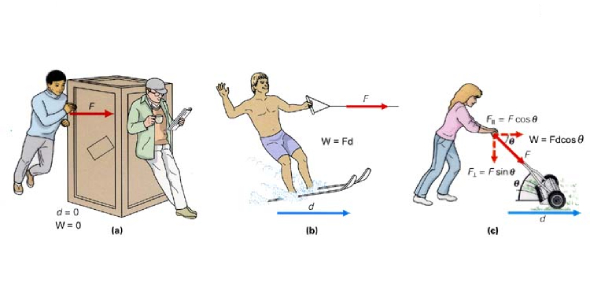# JEE Main Physics: Work And Power Energy Quiz!

10 Questions | Attempts: 725
ShareSettings.

• 1.
A man of mass 60 kg takes 35 seconds to reach the top of a building using the stairs. How much time will be taken by another man of the same mass, if the powers developed by the first man and the second man are in the ratio of 4:7?
• A.

16 seconds

• B.

9 seconds

• C.

18 seconds

• D.

20 seconds

• 2.
Which of the following statement is correct?
• A.

Kinetic Energy of a system can be changed without changing its momentum

• B.

Work done on a system will always increase the potential energy of the system

• C.

Change in the total mechanical energy of the system is equal to the work done by a conservative force

• D.

None of these

• 3.
On a smooth semicircular track of radius ‘R’, a block of mass ‘M’ is given a velocity of . The direction of velocity is parallel to the track at point C. The normal reaction between block and track at point ‘B’ is:
• A.

4Mg

• B.

2Mg

• C.

3Mg

• D.

5Mg

• 4.
An engine pumps water continuously through a nozzle. The water is coming out of the nozzle with a speed ‘v’. At what rate the kinetic energy is imparted by the jet pump to the water jet. Mass per unit length of the water jet is ‘m’.
• A.
• B.
• C.
• D.
• 5.
A car of mass ‘m’ is driven with a constant power ‘P’. What will be the speed of the car after traveling a distance‘s’?
• A.
• B.
• C.
• D.
• 6.
A particle of mass ‘m’ is moving with a variable velocity  where k is a positive constant. If the particle starts from rest, then calculate the work done by all the forces after the particle has travelled a distance ‘x’:
• A.
• B.
• C.
• D.
• 7.
The displacement of an object moving on a straight line varies according to the equation x = Ktn, where t is time. If a constant power is supplied to the object then the value of ‘n’ will be:
• A.

1

• B.

3/2

• C.

1/3

• D.

2

• 8.
A small sphere of mass ‘m’ is released from rest from point A, and the sphere follows the path as shown in the figure. What should be the minimum height ‘H’, so that the sphere is able to complete the circle?
• A.

H = 3/2 R

• B.

H = 5/2 R

• C.

H = 27/2 R

• D.

H = 1/3 R

• 9.
Which of the following graph is the best representation of the kinetic energy of a projectile versus its horizontal displacement?
• A.
• B.
• C.
• D.
• 10.
A particle of mass ‘m’ is moving in horizontal direction on a rough surface. The coefficient of friction varies as , where x is the distance from the origin and K is a positive constant. The particle starts moving from x = 1, in the direction away from the origin. What should be the minimum initial velocity, so that the particle never stops?
• A.
• B.
• C.
• D.

## Related TopicsBack to top
×

Wait!
Here's an interesting quiz for you.